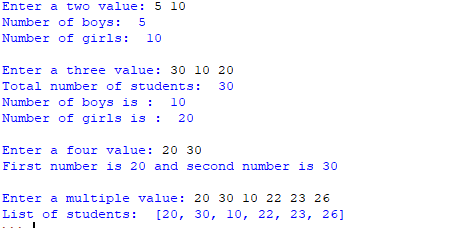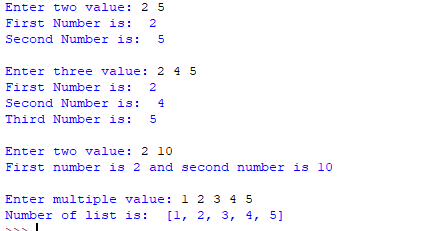Open in App
Not now

# Taking multiple inputs from user in Python

• Difficulty Level : Easy
• Last Updated : 08 Jul, 2022

The developer often wants a user to enter multiple values or inputs in one line. In C++/C user can take multiple inputs in one line using scanf but in Python user can take multiple values or inputs in one line by two methods.

• Using split() method
• Using List comprehension

Using split() method :
This function helps in getting multiple inputs from users. It breaks the given input by the specified separator. If a separator is not provided then any white space is a separator. Generally, users use a split() method to split a Python string but one can use it in taking multiple inputs.

Syntax :

`input().split(separator, maxsplit)`

Example :

## Python3

 `# Python program showing how to ` `# multiple input using split ` ` `  `# taking two inputs at a time ` `x, y ``=` `input``(``"Enter two values: "``).split() ` `print``(``"Number of boys: "``, x) ` `print``(``"Number of girls: "``, y) ` `print``() ` ` `  `# taking three inputs at a time ` `x, y, z ``=` `input``(``"Enter three values: "``).split() ` `print``(``"Total number of students: "``, x) ` `print``(``"Number of boys is : "``, y) ` `print``(``"Number of girls is : "``, z) ` `print``() ` ` `  `# taking two inputs at a time ` `a, b ``=` `input``(``"Enter two values: "``).split() ` `print``(``"First number is {} and second number is {}"``.``format``(a, b)) ` `print``() ` ` `  `# taking multiple inputs at a time  ` `# and type casting using list() function ` `x ``=` `list``(``map``(``int``, ``input``(``"Enter multiple values: "``).split())) ` `print``(``"List of students: "``, x) `

Output:Using List comprehension :
List comprehension is an elegant way to define and create list in Python. We can create lists just like mathematical statements in one line only. It is also used in getting multiple inputs from a user.Example:

## Python3

 `# Python program showing ` `# how to take multiple input ` `# using List comprehension ` ` `  `# taking two input at a time ` `x, y ``=` `[``int``(x) ``for` `x ``in` `input``(``"Enter two values: "``).split()] ` `print``(``"First Number is: "``, x) ` `print``(``"Second Number is: "``, y) ` `print``() ` ` `  `# taking three input at a time ` `x, y, z ``=` `[``int``(x) ``for` `x ``in` `input``(``"Enter three values: "``).split()] ` `print``(``"First Number is: "``, x) ` `print``(``"Second Number is: "``, y) ` `print``(``"Third Number is: "``, z) ` `print``() ` ` `  `# taking two inputs at a time ` `x, y ``=` `[``int``(x) ``for` `x ``in` `input``(``"Enter two values: "``).split()] ` `print``(``"First number is {} and second number is {}"``.``format``(x, y)) ` `print``() ` ` `  `# taking multiple inputs at a time  ` `x ``=` `[``int``(x) ``for` `x ``in` `input``(``"Enter multiple values: "``).split()] ` `print``(``"Number of list is: "``, x)  `

Output :Note: The above examples take input separated by spaces. In case we wish to take input separated by comma (, ), we can use the following:

## Python3

 `# taking multiple inputs at a time separated by comma ` `x ``=` `[``int``(x) ``for` `x ``in` `input``(``"Enter multiple value: "``).split(``","``)] ` `print``(``"Number of list is: "``, x)  `

Please see https://ide.geeksforgeeks.org/BHf0Cxr4mx for a sample run.

My Personal Notes arrow_drop_up
Related Articles